Hello and welcome to our community! Is this your first visit?1. ## Diving Sums

Diving Sums

Nowadays calculations are done by computers, laptops & phones. I know when I did my course the sums behind technical diving (both OC and CCR) was broadly glossed over. For example when I did my CCR course I remember that the equations were shown but mostly just to make the point that a fixed PO2 rather than a fixed FO2 is the main difference between OC and CCR diving.

Thinking back to the OC course we did a few more sums as we needed to figure out what gas to use and from what depth it would be OK. On the blender course we did a few calculations, but mostly we used a look-up-table or a software program.

There are a few other calculations which I've found useful over the years and I'm planning to round them up here in a series of short posts. Here's the list I have ready to go - and I may add more later:

• Gas Selection
• Isobaric Counter Diffusion
• Gas Density
• What gas am I breathing?
• Cylinder Filling for Trimix

I'm a practitioner and not a theorist so some of the formulas are not conventionally formatted – they are intended to be used not studied and therefore are formatted a bit more practically. Also I'm a CCR diver so they all have a CCR slant - but they work the same for OC too.

A note of caution – these calculations are metric. Imperial diving units are just crazy! Also I do not always use depth in meters I sometimes use depth in bar – whatever makes sense in the context of the calculation – here is what I mean: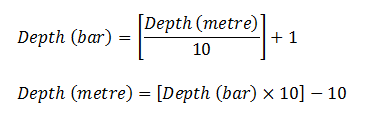I'm not an instructor and there maybe mistakes. If anyone finds one then I'll correct it. There's other ways to arrive at the same answer - I find that although owning and using a computer, laptop and phone is the way I do most things a little bit of theory never hurt and some people might find this useful.

If you don't like sums don't read on :-)

Matt.Reply With Quote

2. Gas Selection

One of the first things to do is to work out what gas we will take on our dive – both for OC bailout and for diluent. Ideally we will create a new mix for every dive using the Ideal Mix equations. More practically we will check that our existing mixes are adequate for our dive. The latter is of course less critical on CCR as the unit blends the actual gas as we go.

Ideal Gas

There’s 2 parts to ideal gas calculation – first the oxygen and then the helium.

Oxygen component is selected based on the desired PO2 at depth:The oxygen component for CCR isn't as important as OC as we do not need to optimise it for decompression but still we must set it to something sensible, here’s an example: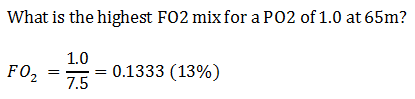Next we can work out the helium requirements based on Equivalent Narcotic Depth (END), END calculates the amount of nitrogen we can tolerate for our selected Equivalent Air Depth as follows, the balance is the helium:Combining this with the oxygen calculation you can figure out your ideal-gas:Is my gas OK for my dive?

More often than not you've already blended your gas before you go diving so all you really care about is making sure it’s OK for the dive selected for the day. This is one of the advantages of CCR diving over OC!

First analyse the gas for oxygen and check the PO2:Here’s an example to see if the analysed gas can be used for a 65m dive:Now mark the cylinder the Maximum Operating Depth (MOD):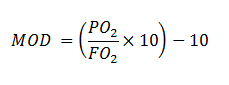He’s an example: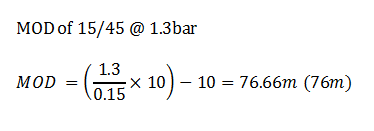Finally we need to check out Equivalent Narcotic Depth (END):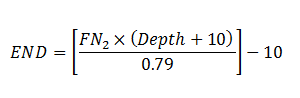Fitting our FN2 and proposed depth into the equation:Reply With Quote

3. A word on Isobaric Counter Diffusion (ICD)

Actually, only a word on the calculation  whether you subscribe to the theory is up to you.

ICD simply checks the PN2 change following a gas switch and deems it safe or unsafe according to a selected threshold. Typically 0.5 is selected as safe although the information is scant.

IDC is only said to be a problem when at or near to a decompression ceiling and most reports seem to imply its only relevant for deep (over 100m) dives.

Its calculated as follows: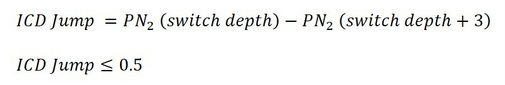IDC calculation takes into account how gasses are used, its clearer with a couple of examples (assuming 3m stop intervals). The first example shows IDC jump greater than 0.5:And if this bothers you then adding some helium brings it under 0.5:Remember to calculate your CCR gas (see below - What gas am I breathing) before working out the PN2.Reply With Quote

4. Gas Density

This part is really only for interest. It shows how to figure out the density of a gas at any depth and then how to compare that to Air to get the Equivalent Air Density.

Gas Density is as follows: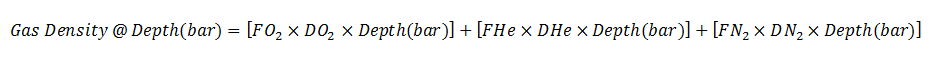To use this we need to know the density of our favourite diving gases. These are: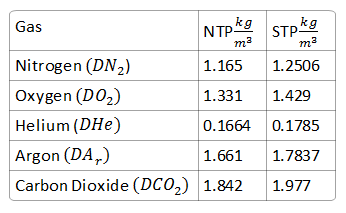The table shows Normal Pressure and Temperature (NPT) and Standard Pressure and Temperature (SPT). Here we use only SPT.So calculating a couple of different densities as an example:OK, so this all seems a bit pointless. However the interesting part is the calculation of Equivalent Air Density:Many academic papers show calculations for things like Work of Breathing based on air. But at what depth can a Trimix be used to get the same effect? What we need to know is the Equivalent Air Density of the Trimix. Lets do an example: assume we want to find the depth at which 15/45 is the same as air at 40m: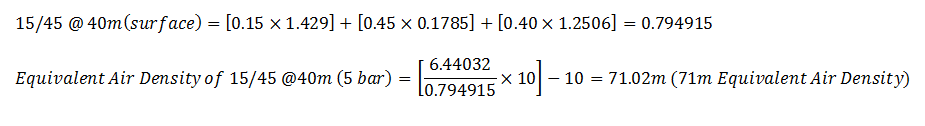Reply With Quote

5. What gas am I breathing?

With OC the gas you are breathing (the fractions) stay the same. Not true with CCR, the PO2 is constant and the FO2 is variable. The inert gasses Helium and Nitrogen take the balance and remain at the same ratio as your diluent. For these calculations I am ignoring expired off-gas (assuming it also to be in the same ratio).

Remember for a given depth and fixed set-point we can calculate FO2: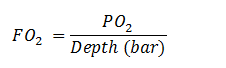For a given diluent we can calculate the inert gases ratio: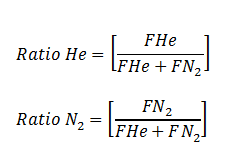From this we can work out the fractions of gas we have:You can simplify the N2 calculation as follows as once you have the He the N2 is the balance:Here’s an example – what gas mix do we have in the loop at 60m using 15/45 and SP=1.3?Reply With Quote

6. It's stuff like this that makes people take up skiing, mountain biking, sofa-hugging etc.Reply With Quote

7. Cylinder Filling for Trimix

Assuming you only have Oxygen, Helium and Air available to you for cylinder filling then how do you go about working out what to put into the cylinder? First you can work out the Helium percentage  thats quite straight forward as it is just the percentage. The oxygen is more tricky as some of it is made from pure O2 and some from the air. Fortunately we remember how to make Nitrox (EANx) and Trimix is just a Nitrox with Helium: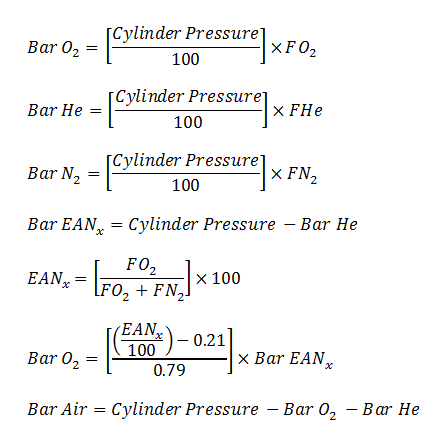For an empty cylinder we just plumb our numbers in once and we have our fill-formula. But what do we do to make a top-up? Top-up means we have to run the calculation twice  once for the gas we currently have (the Got) and again for what we want (the Want). Then we can work-out the difference:Its easier with an example. Lets assume we have 120 bar of 11/52 and we want to convert it to 230 bar of 15/45. First work out what we have got: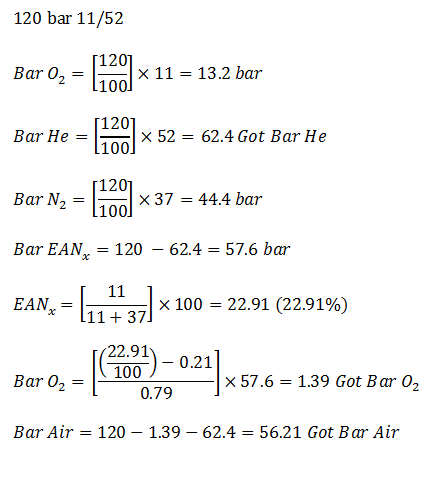Then what we want:Then the subtractions:The final filling instruction is:Reply With Quote

8. Nice one nickReply With Quote

9.Originally Posted by Nitnab NohjIt's stuff like this that makes people take up skiing, mountain biking, sofa-hugging etc.
Trust BJ to make a sarky post right in the middle of things, lol.

HNY John.

Matt.Reply With Quote

10. Nice.
If you're happy always thinking of depth in atmospheres, you can make END & best mix even simpler, and allow for both O2 narcotic & non-narcotic. Here's what I use:

END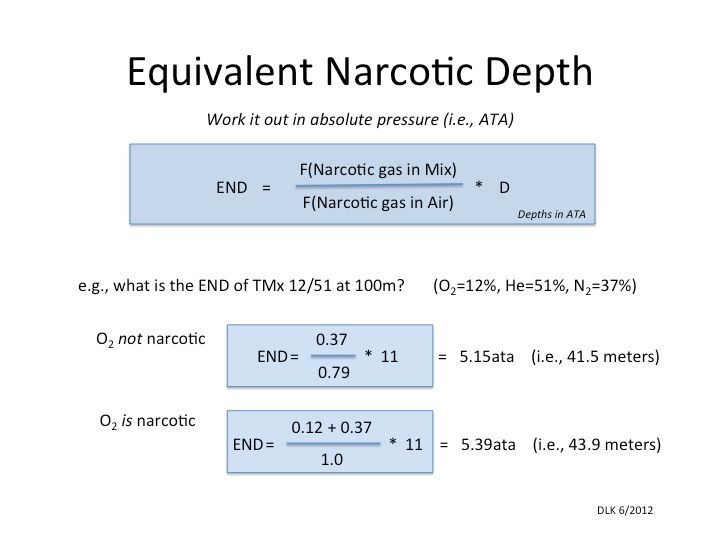And Best Mix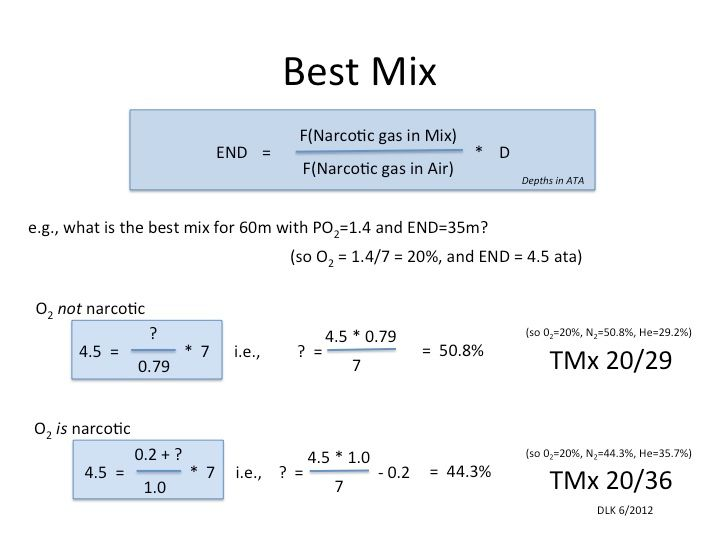With a bit of jiggery-pokery, you can also use these to 'equivalize' ENDs... e.g., What mix should I dive at 90m if I want the same END as a 20/30 at 50m.Reply With Quote

####Posting Permissions

• You may not post new threads
• You may not post replies
• You may not post attachments
• You may not edit your posts
•